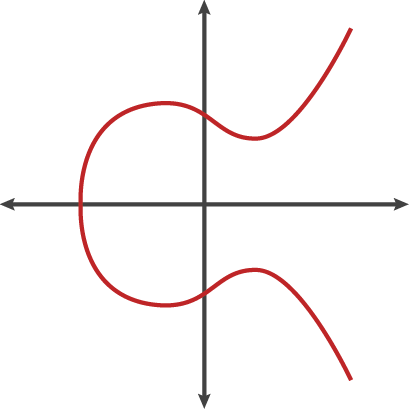Understanding btcd - Part 2: Key and Address
The btcec package implements elliptic curve cryptography, helps you working with bitcoin public, private keys and addresses. It's also the algorithm needed to sign and verify data.

So how do you create a private, public key-pair?

### Create private, public key-pair

The thing you should know is that public key can be generated from the private key. So having the private key is equivalent to having the whole key-pair.

This is the structure of a key-pair in btcd:

``````func PrivKeyFromBytes(curve elliptic.Curve, pk []byte) (*PrivateKey, *PublicKey) {
x, y := curve.ScalarBaseMult(pk)
priv := &ecdsa.PrivateKey{
PublicKey: ecdsa.PublicKey{
Curve: curve,
X:     x,
Y:     y,
},
D: new(big.Int).SetBytes(pk),
}
return (*PrivateKey)(priv), (*PublicKey)(&priv.PublicKey)
}``````

An *ecdsa.PrivateKey is a struct of PublicKey and PrivateKey. That’s also the function to retrieve a key-pair from raw bytes PrivateKey.

So you would know how to generate a new key-pair. Just randomize the private key:

``````func NewPrivateKey(curve elliptic.Curve) (*PrivateKey, error) {
key, err := ecdsa.GenerateKey(curve, rand.Reader)
if err != nil {
return nil, err
}
return (*PrivateKey)(key), nil
}``````

What is the elliptic.Curve in the function above? It’s the parameters belong to eliptic curve of ECDSA algorithm. Currently, Bitcoin uses secp256k1. And there are many more curve parameters which differ in properties, such as speed, efficiency,… (http://www.secg.org/sec2-v2.pdf)This is an elliptic curve. You can see PublicKey is ecdsa.PublicKey{ Curve: curve, X: x, Y: y} is a point in the curve.

### Bitcoin Addresses

Bitcoin address is the PublicKey of the key-pair. But instead of an array of bytes, the public key is created by hashing the PublicKey, adding more checksum,… The purpose of this is making the address easier reading for human, error checking and harder to be mistakenly typed.

This is the process of getting the address from a PublicKey:So the address of Bitcoin contains three parts: Version, Public Key Hash, and Checksum. The code to transform PublicKey into address is as below:

``````func checksum(payload []byte) []byte{
firstSHA := sha256.Sum256(payload)
secondSHA := sha256.Sum256(firstSHA[:])
return secondSHA[:4]
}

func HashPubKey(pubKey []byte) []byte{
publicSHA256 := sha256.Sum256(pubKey)
RIPEMD160Hasher := ripemd160.New()
_, err := RIPEMD160Hasher.Write(publicSHA256[:])
if err != nil{
log.Panic(err)
}
publicRIPEMD160 := RIPEMD160Hasher.Sum(nil)
return publicRIPEMD160

}

func getAddress(pubKey []byte) []byte{
pubKeyHash := HashPubKey(pubKey)
versionedPayload := append([]byte{version}, pubKeyHash...)
checksum :=  checksum(versionedPayload)
fullpayload := append(versionedPayload, checksum...)
address := Base58Encode(fullpayload)
return address
}``````

One more thing, public key is a point on elliptic curve. In Bitcoin, the curve reflects itself between X-axis, so there are 2 ways of representing Bitcoin address:

1. Uncompressed address, which involves all (X, Y) information

2. Compressed address, which involves (X) and Y should be determined by the point is above or below X-axis.

A compressed key is just a way of storing a public key in fewer bytes (33 instead of 65). They are the same keys, which point to the same point on the curve, just stored in a different way. With a compressed address, the transaction will be a bit smaller, resulting in a bit smaller fee.

So how do we get compressed key? Of course, we only use publickey.X coordinate, and determines publicKey.Y is on which side.

``````func isOdd(b *big.Int) bool{
if b.Bit(0) == 0 {
return false
} else {
return true
}
}

func getCompressedPubKey(publicKey *ecdsa.PublicKey) string{
var compressedPub []byte

if isOdd(publicKey.Y){
compressedPub =  append([]byte{0x03}, publicKey.X.Bytes()...)
}else{
compressedPub =  append([]byte{0x02}, publicKey.X.Bytes()...)
}
return key
}``````

### Sign and Verify data

Sign and Verify takes a very important role in how Bitcoin works. This cryptography ensures the validity and untampered of data, mostly in Bitcoin transactions. As a system working with money, this is a critical feature.

With a public and private key-pair, we can sign and verify data as below:

``````message := `test message`;
messageHash := chainhash.DoubleHashB([]byte(message))
signature, err := privKey.Sign(messageHash)``````
``verified := signature.Verify(messageHash, pubKey)``

You can see how it works here: We use a PrivateKey to calculate the Signature of a message, then only the Signature and PublicKey is needed to verify it. That’s the core feature of the Elliptic Curve Digital Signature Algorithm.

### Refercences

You can play with the source code of this tutorial at https://github.com/hlongvu/golang_ecdsa/blob/master/main.go

There are tools for online testing key-pair generation:

1. Test private key to public key here:

Some helpful documents about Compressed and Uncompressed Addresses

1. https://bitcoin.stackexchange.com/questions/41662/on-public-keys-compression-why-an-even-or-odd-y-coordinate-corresponds-to-the-p

2. https://bitcoin.stackexchange.com/questions/69315/how-are-compressed-pubkeys-generated

> Look at the value of the y-coordinate. If the last digit is an odd number (1, 3, 5, 7, 9) then use a prefix of “03”. If it is not an odd number (0, 2, 4, 6, 8 ) then use a prefix of “02”

3. https://bitcointalk.org/index.php?topic=2185929.msg21939806#msg21939806

4. https://bitcoin.stackexchange.com/questions/3059/what-is-a-compressed-bitcoin-key